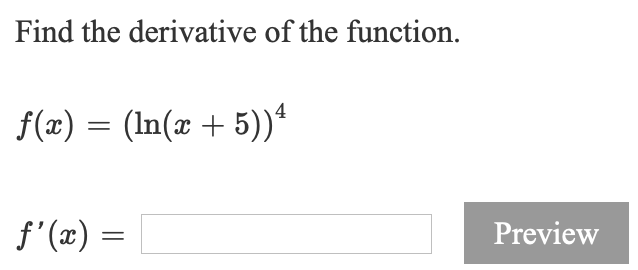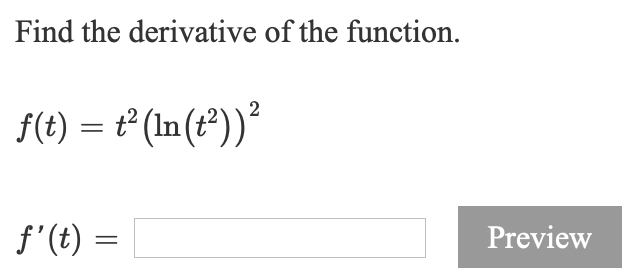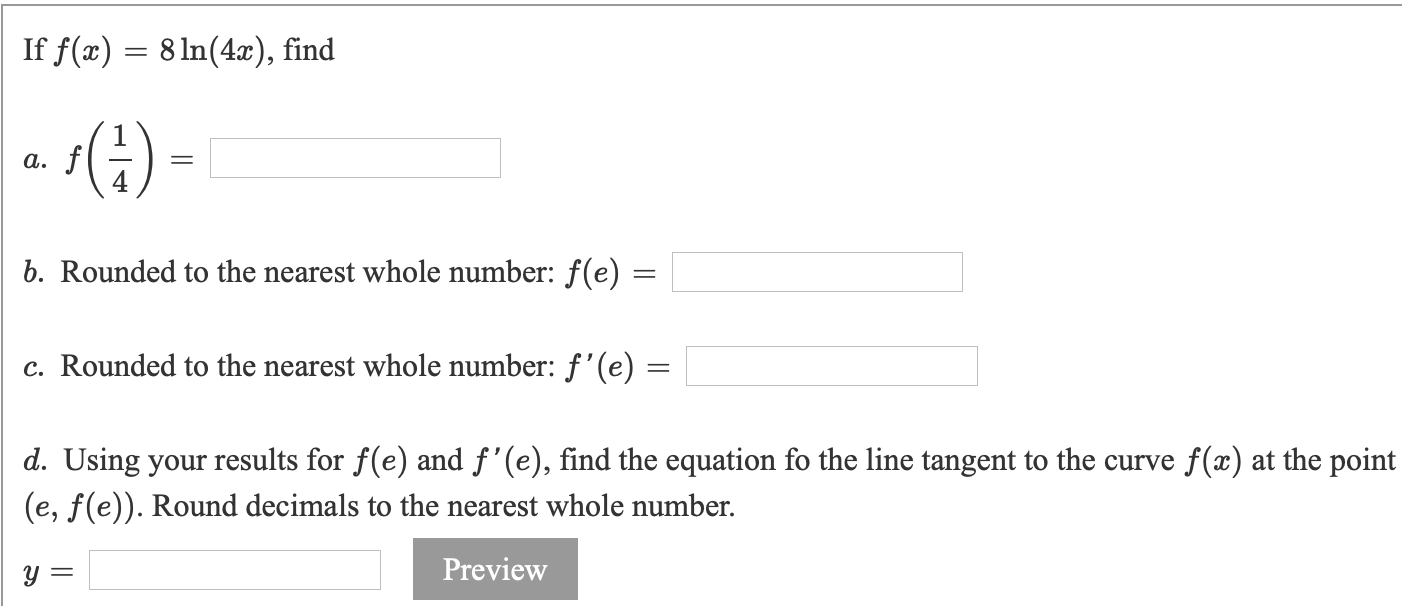# Find the derivative of the function. f(x) = (ln(x + 5)) f'(c) = Preview Find the...

###### Question:Find the derivative of the function. f(x) = (ln(x + 5)) f'(c) = Preview
Find the derivative of the function. f(t) = ť(In(t))? f'(t) = Preview
If f(a) = 8 ln(4x), find a. f b. Rounded to the nearest whole number: f(e) c. Rounded to the nearest whole number: f'(e) = d. sing your results for f(e) and f'(e), find the equation fo the line tangent to the curve f(x) at the point (e, f(e)). Round decimals to the nearest whole number. y = Preview

#### Similar Solved Questions

Please show all steps and work please You are assigned to follow up on purchases made through your company’s catalog mailing. You find that 10% ordered a hat and you also find that given someone ordered a hat, 60% also ordered mittens. You also note that 20% of the people who did not order a h...